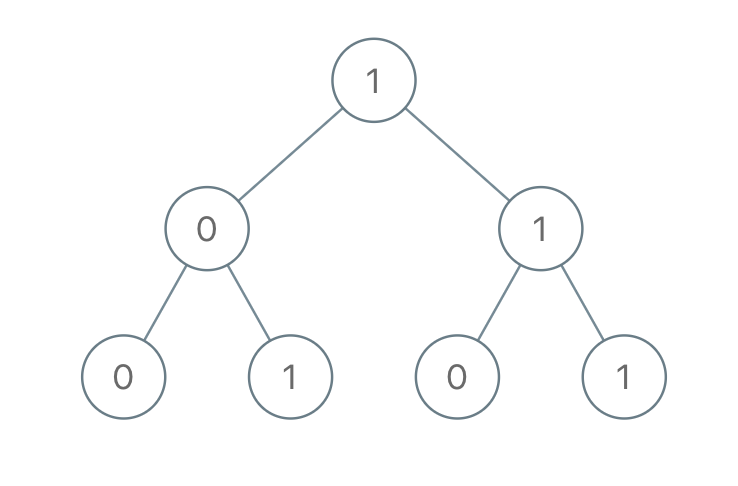Formatted question description: https://leetcode.ca/all/1022.html

# 1022. Sum of Root To Leaf Binary Numbers (Easy)

Given a binary tree, each node has value 0 or 1.  Each root-to-leaf path represents a binary number starting with the most significant bit.  For example, if the path is 0 -> 1 -> 1 -> 0 -> 1, then this could represent 01101 in binary, which is 13.

For all leaves in the tree, consider the numbers represented by the path from the root to that leaf.

Return the sum of these numbers.

Example 1:Input: [1,0,1,0,1,0,1]
Output: 22
Explanation: (100) + (101) + (110) + (111) = 4 + 5 + 6 + 7 = 22


Note:

1. The number of nodes in the tree is between 1 and 1000.
2. node.val is 0 or 1.
3. The answer will not exceed 2^31 - 1.

Companies:
Amazon

Related Topics:
Tree

## Solution 1.

// OJ: https://leetcode.com/problems/sum-of-root-to-leaf-binary-numbers/

// Time: O(N)
// Space: O(H)
class Solution {
int ans = 0;
void preorder(TreeNode *root, int path) {
if (!root) return;
path = (path << 1) + root->val;
if (!root->left && !root->right) {
ans += path;
return;
}
preorder(root->left, path);
preorder(root->right, path);
}
public:
int sumRootToLeaf(TreeNode* root) {
preorder(root, 0);
return ans;
}
};


Or

// OJ: https://leetcode.com/problems/sum-of-root-to-leaf-binary-numbers/

// Time: O(N)
// Space: O(H)
class Solution {
int sum = 0;
public:
int sumRootToLeaf(TreeNode* root, int path = 0) {
if (!root) return 0;
path = (path << 1) + root->val;
if (!root->left && !root->right) sum += path;
else {
sumRootToLeaf(root->left, path);
sumRootToLeaf(root->right, path);
}
return sum;
}
};


Java

/**
* Definition for a binary tree node.
* public class TreeNode {
*     int val;
*     TreeNode left;
*     TreeNode right;
*     TreeNode(int x) { val = x; }
* }
*/
class Solution {
public int sumRootToLeaf(TreeNode root) {
int sum = 0;
nodeQueue.offer(root);
numQueue.offer(root.val);
while (!nodeQueue.isEmpty()) {
TreeNode node = nodeQueue.poll();
int num = numQueue.poll();
TreeNode left = node.left, right = node.right;
if (left == null && right == null)
sum += num;
else {
if (left != null) {
nodeQueue.offer(left);
numQueue.offer(num * 2 + left.val);
}
if (right != null) {
nodeQueue.offer(right);
numQueue.offer(num * 2 + right.val);
}
}
}
return sum;
}
}